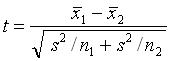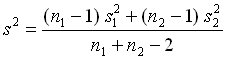Search BioKin.comTwo treatment groups
Statistical calculator for the difference in two treatment groupsThe Java applet contained in this page will compute the probability, p, that the difference in treatment between two groups is not statistically significant.

The hypothesis being tested is that the two samples (groups) come from the same distribution. A low value of probability p (for example, p < 0.05) means that this null-hypothesis should be rejected. In other words, low p means that there is significant difference between the two groups being compared.

#### Method

Assume that two samples were drawn, with sample sizes n1 and n2, and that some quantity was measured for each member of the particular data set. The mean values of the measured quantity in each sample are x1 and x2, and the corresponding standard deviations are s1 and s2.

The Student-tstatistic is calculated by using equation (1), where the pooled variance s2 is defined as shown in equation (2).(1)(2)

This calculator will compute the probability p that the Student-t statistic computed from the input data is large enough to reject the hypothesis that the two samples were drawn from the same distribution (i.e., that any difference in sample means is purely due to random chance).

#### Example 1

T. J. Conahan et al. reported in the journal Anesthesiology 38:528-535 (1973) the "best cardiac index" measured for two groups of patients. The first group of nine patients received Halothane as an anesthetic while the second group of sixteen patients received Morphine. The mean + standard deviation cardiac index was 2.08 + 1.05 for the Halothane group and 1.75 + 0.88 for the Morphine group. Is there a signicant difference between the two treatments?

To answer this question, type the following values into the input data form:

 1. mean 2.08 1. standard deviation 1.05 1. sample size 9 2. mean 1.75 2. standard deviation 0.88 2. sample size 16

Press the COMPUTE button to obtain the following result:

 probability 0.409

This value is higher than the conventional critical value of p = 0.05, which means that at the 95% confidence level we cannot reject accept the hypothesis that the samples were drawn from the same population. In other words, there is not a significant difference between administering either Halothane or Morphine.

#### Reference

Stanton A. Glanz (1992) Primer of Biostatistics, 3rd Ed., McGraw-Hill, New York, pp. 81-88.
[ISBN 0-07-023511-2]www.biokin.com/tools/twogroups.html
Sat Apr 20 18:22:32 2019